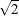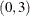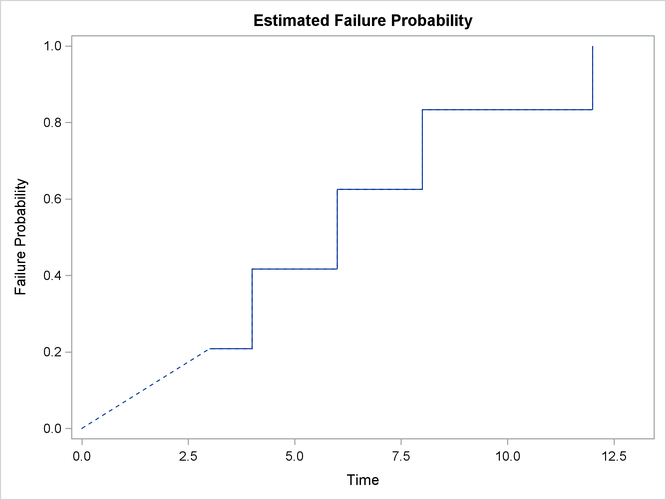# The ICLIFETEST Procedure

### Example 62.1 Analyzing Data with Observations below a Limit of Detection

Data that have certain values below a limit of detection (LOD) are frequently encountered by toxicologists and environmental scientists. Such data are usually analyzed by imputing the unobserved values by LOD/2 or LOD/. This type of practice often raises the question of whether the population distributions can be estimated without bias. Gillespie et al. (2010) propose using a reverse Kaplan-Meier estimator, or equivalently, Turnbull’s method (1976) by treating the unobserved data as left-censored. When the assumption of independent censoring holds, these estimators can unbiasedly estimate the population distribution functions.

The following hypothetical data have two values, 3 and 10, that are below the limit of detection:

data temp;
input C1 C2;
datalines;
.     3
4     4
6     6
8     8
.     10
12    12
;


The following statements invoke PROC ICLIFETEST to estimate the population distribution function by using Turnbull’s method:

proc iclifetest data=temp method=turnbull plots=survival(failure)
impute(seed=1234);
time (c1,c2);
run;


Specifying the PLOTS=SURVIVAL(FAILURE) option requests a failure probability plot. Results are shown in Output 62.1.1. Note that because the first Turnbull interval is, the failure probability function is undefined within that interval.

Output 62.1.1: Failure Probability Plot for Fictitious Nondetection DataOutput 62.1.2 presents the estimated failure probability, with standard errors that are estimated by the method of multiple imputations.

Output 62.1.2: Cumulative Probability Estimates

The ICLIFETEST Procedure

Nonparametric Survival Estimates
Probability Estimate Imputation
Standard
Error
Lagrange
Multiplier
Time Interval Failure Survival
3 4 0.2083 0.7917 0.1811 0.0000
4 6 0.4167 0.5833 0.2179 0.0000
6 8 0.6250 0.3750 0.2099 0.0000
8 12 0.8333 0.1667 0.1521 0.0000
12 Inf 1.0000 0.0000 0.0000 0.0000